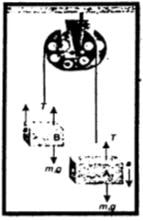Calculate the tension and acceleration in the string during the motion of bodies connected by the string and passing over the friction pulley using the second law of motion?

Difficulty: Hard

Vertical motion of two bodies attached to the ends of a string that passes over the frictionless pulley:

Suppose two bodies A and B having masses m1 and m2 respectively are connected to two ends of an inextensible string that passes over a frictionless pulley. If m1 is greater than m2, then body A will move downward and body B will move upward.

Two forces are acting on the body A:

1. its weight $w_{1} = m_{1}g$
2. Tension on string _{T1}

Since body A will move downward, hence its weight m1g is greater than the tension T in the string.

The net force acting on body$A =m _{1}g$

According to Newton’s second law of motion:

$m _{1}g - T = m _{1} a$ ......(1)

Two forces are acting on the body B:

1. Its weight  $w _{2}= m _{2}g$ acting downward
2. Tension of string T acting upward.

As the body moves upward, hence its weight m2g is less than the tension T in the string

Net force acting on body $B = T - m _{2}g$

According to Newton’s second law of motion:

$T - m _{2}g = m _{2}a$ ........(2)

Adding Eq. (i) and Eq. (ii), we get acceleration a.$m_{1}g - m _{2}g = m _{1}a + m _{1}a$

$\left(m_{1} - m_{2}\right)a =\left(m_{1} +m _{2}\right)a$

Magnitude of acceleration = $a=\frac{m_{1}-m_{2}}{m_{1}+ m _{2}} g$ .......(3)

Putting the valueanof a in Eq. (ii), we get

$T -m_{2}g =m_{2}\left(\frac{m_{1}-m_{2}}{m_{1}+ m _{2}} g\right)$

$T = m{2}g + m_{2}\left(\frac{m_{1}-m_{2}}{m_{1}+ m _{2}} g\right)$

$T = m{2}g\left[1 + \frac{m_{1}-m_{2}}{m_{1}+ m _{2}}\right]$

$T = m{2}g\left[ \frac{m{1}+ m{2}+m _{1}-m_{2} }{m_{1}+ m _{2}}\right]$

Magnitude of tension = $T =\frac{{2m_{1}} m_{2} }{m_{1}+ m _{2}}g$

Note:

Atwood machine:

The above arrangement is also known as the Atwood machine. It can be used to find the acceleration g due to gravity using Eq. (iii)

$g =\frac{{m_{1}}- m_{2} }{m_{1}+ m _{2}}a$

DO YOU KNOW

An Atwood machine is an arrangement of two objects of unequal masses. Both the objects are attached to the ends of a string. The string passes over a frictionless pulley. This arrangement is sometimes used to find the acceleration due to gravity.